# Samacheer Kalvi 8th Maths Solutions Term 2 Chapter 4 Information Processing Ex 4.2

Students can Download Maths Chapter 4 Information Processing Ex 4.2 Questions and Answers, Notes Pdf, Samacheer Kalvi 8th Maths Book Solutions Guide Pdf helps you to revise the complete Tamilnadu State Board New Syllabus and score more marks in your examinations.

## Tamilnadu Samacheer Kalvi 8th Maths Solutions Term 2 Chapter 4 Information Processing Ex 4.2

Question 1.

Question (i)
Common prime factors of 30 and 250 are
(a) 2 x 5
(b) 3 x 5
(c) 2 x 3 x 5
(d) 5 x 5
(a) 2 x 5
Hint:
Prime factors of 30 are 2 x 3 x 5
Prime factors of 250 are 5 x 5 x 5 x 2
∴ Common prime factors are 2 x 5Question (ii)
Common prime factors of 36, 60 and 72 are
(a) 2 x 2
(b) 2 x 3
(c) 3 x 3
(d) 3 x 2 x 2
(d) 3 x 2 x 2
Hint:
Prime factors of 36 are 2 x 2 x 3 x 3
Prime factors of 60 are 2 x 2 x 3 x 5
Prime factors of 72 are 2 x 2 x 2 x 3 x 3
∴ Common prime factors are 2 x 2 x 3

Question (iii)
Two numbers are said to be co-prime numbers if their HCF is –
(a) 2
(b) 3
(c) 0
(d) 1
(d) 1

Question 2.
Using repeated division method find HCF of the following:

1. 455 and 26
2. 392 and 256
3. 6765 and 610
4. 184, 230 and 276

1. 455 and 26 divisor
Solution:

• Step 1: The larger number should be dividend = 455 & smaller number should be divisor = 26
• Step 2: After 1st division, the remainder becomes new divisor & the previous divisor
• becomes next dividend.
• Step 3: This is done till remainder is zero.
• Step 4: The last divisor is the HCF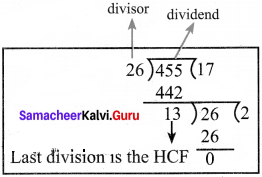The HCF is 13.

2. 392 and 256
256 is smaller, so it is the 1st divisor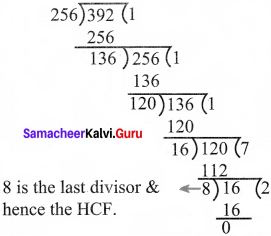∴ HCF = 8

3. 6765 and 610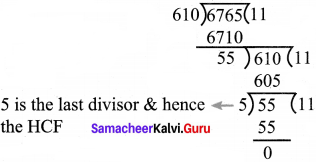∴ HCF = 5

4. 184, 230 and 276
First let us take 184 & 230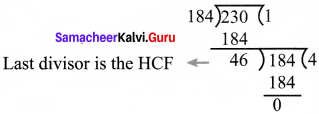∴ 46 is the Hi of 184, and 230.
Now the HCF of the first two numbers is the dividend for the third number.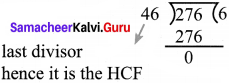HCF of 184, 230 & 276 is 46Question 3.
Using repeated subtracting method find HCF of the following:

1. 42 and 70
2. 36 and 80
3. 280 and 420
4. 1014 and 654

1. 42 and 70
Solution:
Let number be m & n
m > n
We do m – n & the result of subtraction becomes new ‘m’. if m becomes less than n, we do n – m and then assign the result as n. We should do this till m = n. When m = n then ‘m’ is the HCF.
42 and 70 now m = 70, n = 42
70 – 42 = 28, now m = 42, n = 28
42 – 28 = 14, now m = 28, n = 14
28 – 14= 14, now m = 14, n = 14
we stop here as m = n
∴ HCF of 42 & 70 is 14

2. 36 and 80
Solution:
36 and 80 m = 80, n = 36
80 – 36 = 44, now n = 44, m = 36
Since n > m, we should do n m
44 – 36 = 8, now n = 8, m = 36
36 – 8 = 28
Similarly, processing, proceeding, we do repeated subtraction
till m = n
28 – 8 = 20
20 – 8 = 12
12 – 8 = 4
8 – 4 = 4 now m = n = 4
∴ HCF is 4

3. 280 and 420
Solution:
Let m = 420, n = 280
m – n = 420 – 280 = 140
now m = 280, n = 140
m – n = 280 – 140 = 140
now m = n = 140
∴ HCF is 140

4. 1014 and 654
Solution:
Let m = 1014, n = 654
m – n = 1014 – 654 = 360
now m = 654, n = 360
m – n = 654 – 360 = 294
now m = 360, n = 294
m – n = 360 – 294 = 66
now m = 294, n = 66
m – n = 294 – 66 = 228
now m = 66, n = 228
n – m = 228 – 66 = 162
now m = 162, n = 66
∴ m – n = 162 – 66 = 96
n – m = 96 – 66 = 30
Similarly 66 – 30 = 36
36 – 30 = 6
30 – 6 = 24
24 – 6 = 18
18 – 6 = 12
12 – 6 = 6
now m = n
∴ HCF of 1014 and 654 is 6

Question 4.
Do the given problems in repeated subtraction method
1. 56 and 12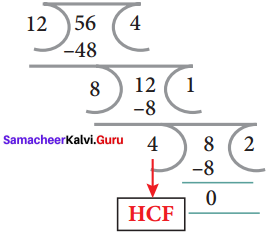2. 320,120 and 95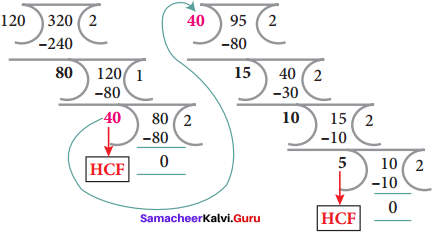1. 56 and 12
Solution:
56 & 12
Let n = 56 & n = 12
m – n = 56 – 12 = 44
now m = 44, n = 12
m – n = 44 – 12 = 32
m – n = 32 – 12 = 20
m – n = 20 – 12 = 8
n – m = 12 = 8 = 4
m – n = 8 – 44. now m = n
HCF of 56 & 12 is 4

2. 320, 120 and 95
Let us take 320 & 120 first m = 320, n = 120
m – n = 320 – 120 = 200
m = 200, n = 120
∴ m – n = 200 – 120 = 80
120 – 80 = 40
80 – 40 = 40
∴ m = w = 40 → HCF of 320, 120
Now let us find HCF of 40 & 95
m = 95, n = 40
∴ m – n = 95 – 40 = 55
55 – 40 = 15
40 – 15 = 25
25 – 15 = 10
15 – 10 = 5
HCF of 40 & 95 is 5 10 – 5 = 5
HCF of 320, 120 & 95 is 5Question 5.
On a school trip, 56 girls and 98 boys went to Kanyakumari. They were divided into as many groups as possible so that there were equal numbers of girls and boys in each group. Find the largest group possible? (To find the HCF using repeated division method)
Solution:
56 girls & 98 boys. Now, we need to find HCF of 56 & 98
Using repeated division method. So, we first divide. 98 by 56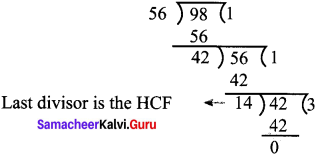14.

Question 6.
Kalai wants to cut identical squares as big as she can, from a piece of paper measuring 168 mm and by 196 mm. What is the length of the side of the biggest square? (To find HCF using repeated subtraction method)
Solution:
Sides are 168 & 196
To find HCF of 168 & 196, we are to use repeated subtraction method.
m = 196, n = 168
m – n = 196 -168 = 28 now n = 28, m = 168
m – n = 168 – 28 = 140 now m = 140, n = = 28
m – n = 140 – 28 = 112 now m = 112, n = 28
m – n = 112 -28 = 84 now m = 84, n = 28
m – n = 84 – 28 = = 56 now m = 56, n = 28
m – n = 56 – 28 = 28
∴ HCF is 28
∴ Length of biggest square is 28